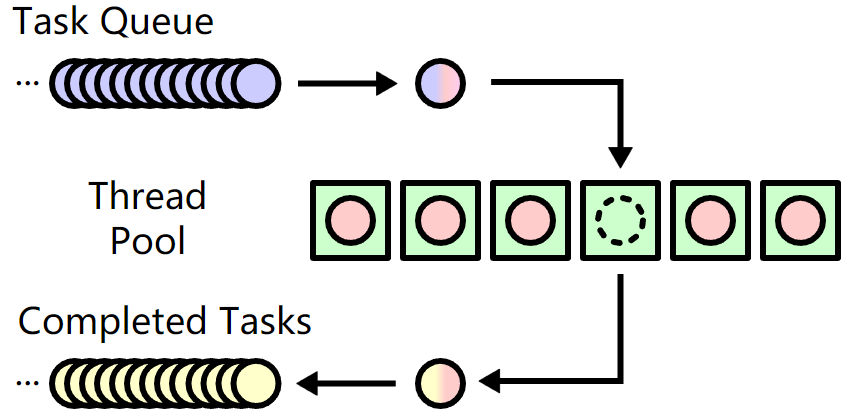# Go基础系列：Go实现工作池的两种方式

## worker pool简介

worker pool中包含了一堆的线程(worker，对go而言每个worker就是一个goroutine)，这些线程嗷嗷待哺，等待着为它们分配任务，或者自己去任务队列中取任务。取得任务后更新任务队列，然后执行任务，并将执行完成的任务放进已完成队列。## 传统互斥锁机制的工作池

type Task struct {
...
}


type Queue struct{
M     sync.Mutex
}


func Worker(queue *Queue) {
for {
// Lock()和Unlock()之间的是critical section
queue.M.Lock()
// 取出任务
// 更新任务队列
queue.M.Unlock()
// 在此goroutine中执行任务
}
}


## 通过buffered channel实现工作池

• goroutine执行任务后，将结果写入到result buffered channel中
• 从result buffered channel中取出计算结果并输出

type Task struct {
ID      int
randnum int
}

type Result struct {
result  int
}

var results = make(chan Result, 10)


func process(num int) int {
sum := 0
for num != 0 {
digit := num % 10
sum += digit
num /= 10
}
time.Sleep(2 * time.Second)
return sum
}


func worker(wg *WaitGroup){
defer wg.Done()
results <- result
}
}


func createWorkerPool(numOfWorkers int) {
var wg sync.WaitGroup
for i := 0; i < numOfWorkers; i++ {
go worker(&wg)
}
wg.Wait()
close(results)
}


func allocate(numOfTasks int) {
for i := 0; i < numOfTasks; i++ {
randnum := rand.Intn(999)
}
}


func getResult(done chan bool) {
for result := range results {
}
done <- true
}


getResult()中使用了一个done参数，这个参数是一个信号通道，用来表示results中的所有结果都取出来并处理完成了，这个通道不一定要用bool类型，任何类型皆可，它不用来传数据，仅用来返回可读，所以上面直接close(done)的效果也一样。通过下面的main()函数，就能理解done信号通道的作用。

func main() {
// 记录起始终止时间，用来测试完成所有任务耗费时长
startTime := time.Now()

numOfWorkers := 20
// 创建任务到任务队列中
// 创建工作池
go createWorkerPool(numOfWorkers)
// 取得结果
var done = make(chan bool)
go getResult(done)

// 如果results中还有数据，将阻塞在此
// 直到发送了信号给done通道
<- done
endTime := time.Now()
diff := endTime.Sub(startTime)
fmt.Println("total time taken ", diff.Seconds(), "seconds")
}


package main

import (
"fmt"
"math/rand"
"sync"
"time"
)

id      int
randnum int
}
type Result struct {
result int
}

var results = make(chan Result, 10)

func process(num int) int {
sum := 0
for num != 0 {
digit := num % 10
sum += digit
num /= 10
}
time.Sleep(2 * time.Second)
return sum
}
func worker(wg *sync.WaitGroup) {
defer wg.Done()
results <- result
}
}
func createWorkerPool(numOfWorkers int) {
var wg sync.WaitGroup
for i := 0; i < numOfWorkers; i++ {
go worker(&wg)
}
wg.Wait()
close(results)
}
for i := 0; i < numOfTasks; i++ {
randnum := rand.Intn(999)
}
}
func getResult(done chan bool) {
for result := range results {
}
done <- true
}
func main() {
startTime := time.Now()
numOfWorkers := 20

var done = make(chan bool)
go getResult(done)
go createWorkerPool(numOfWorkers)
// 必须在allocate()和getResult()之后创建工作池
<-done
endTime := time.Now()
diff := endTime.Sub(startTime)
fmt.Println("total time taken ", diff.Seconds(), "seconds")
}


Task id 19, randnum 914 , sum 14
Task id 9, randnum 150 , sum 6
Task id 15, randnum 215 , sum 8
............
Task id 97, randnum 315 , sum 9
Task id 99, randnum 641 , sum 11
total time taken  10.0174705 seconds


posted @ 2018-11-22 23:12  骏马金龙  阅读(...)  评论(...编辑  收藏